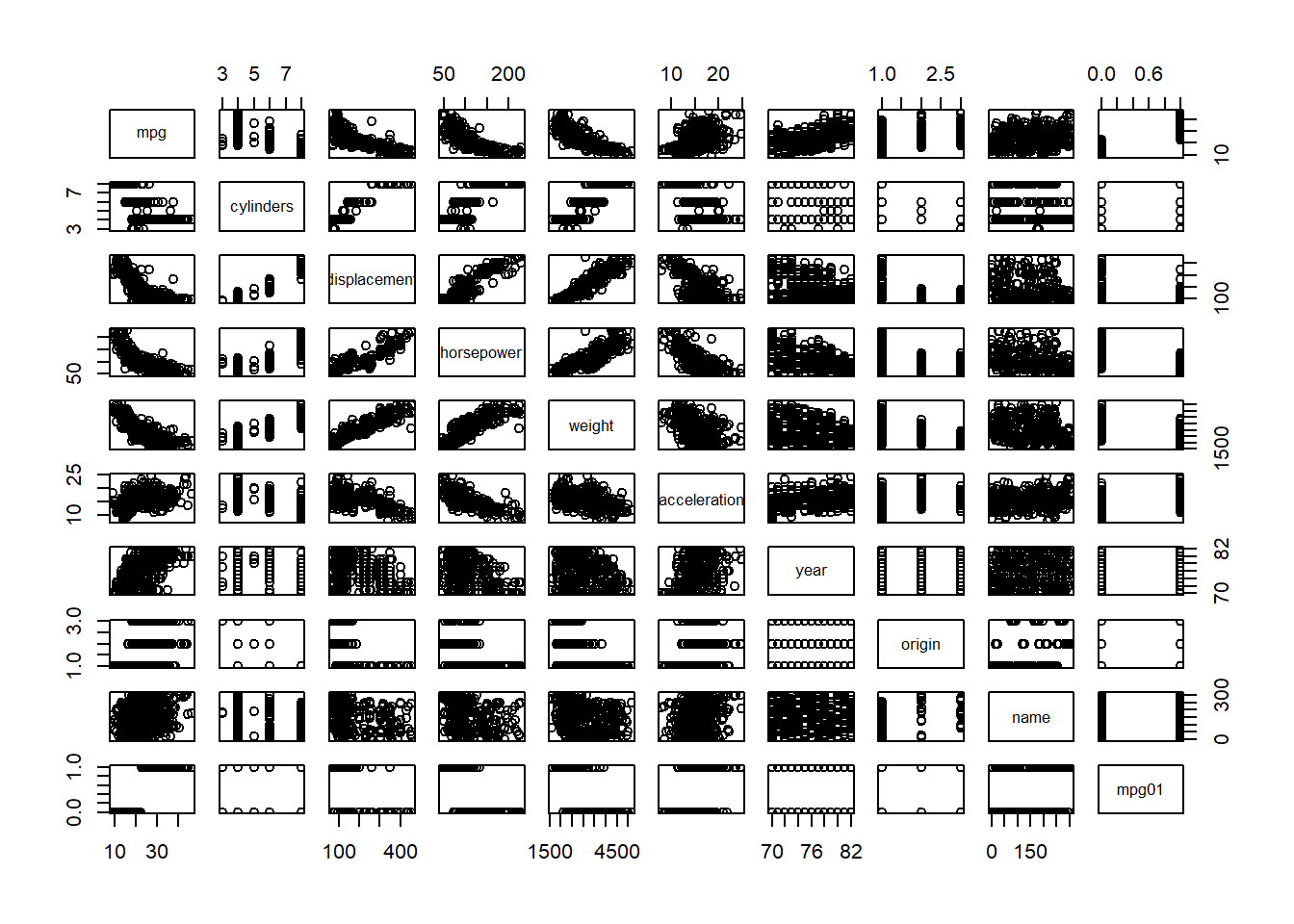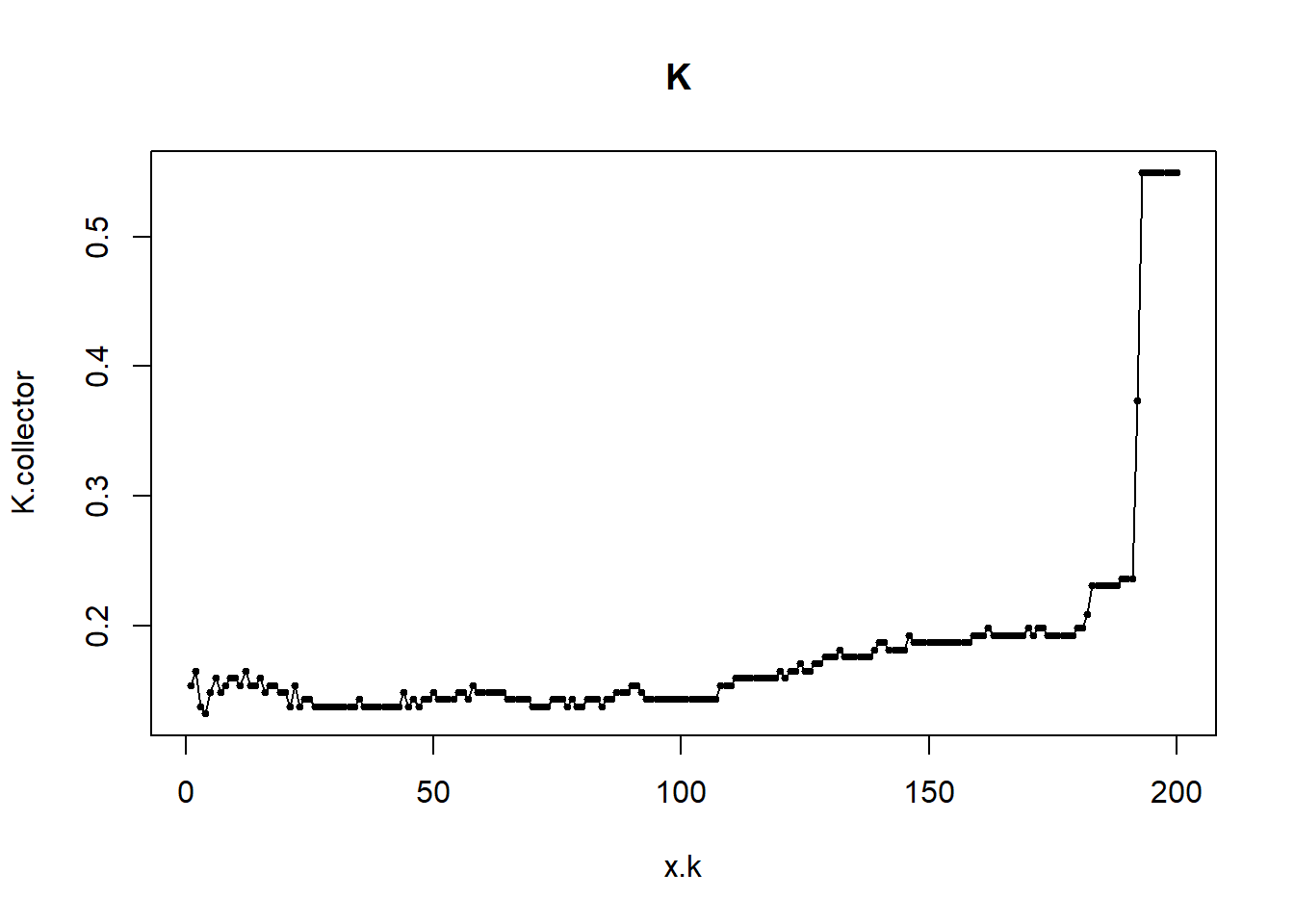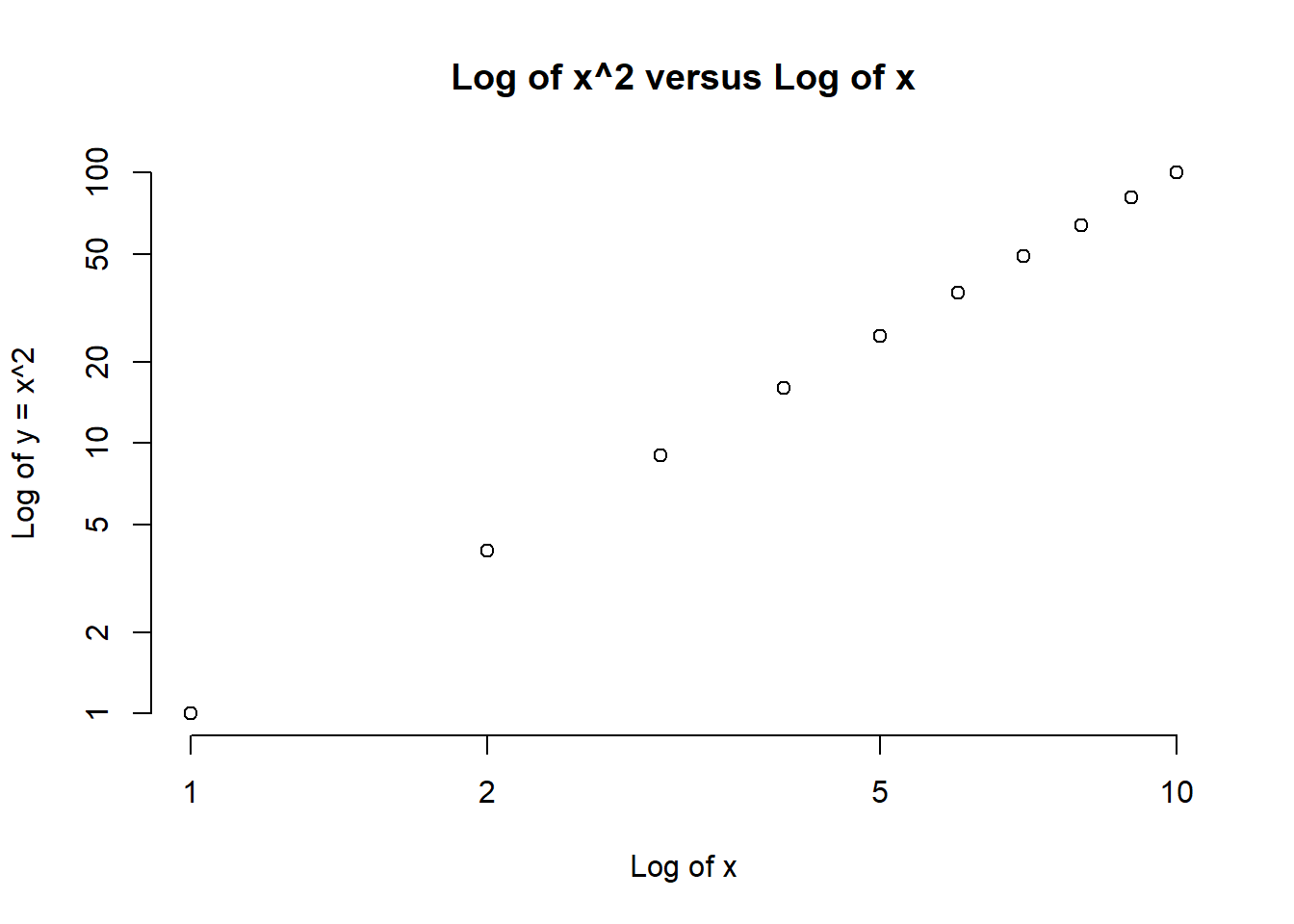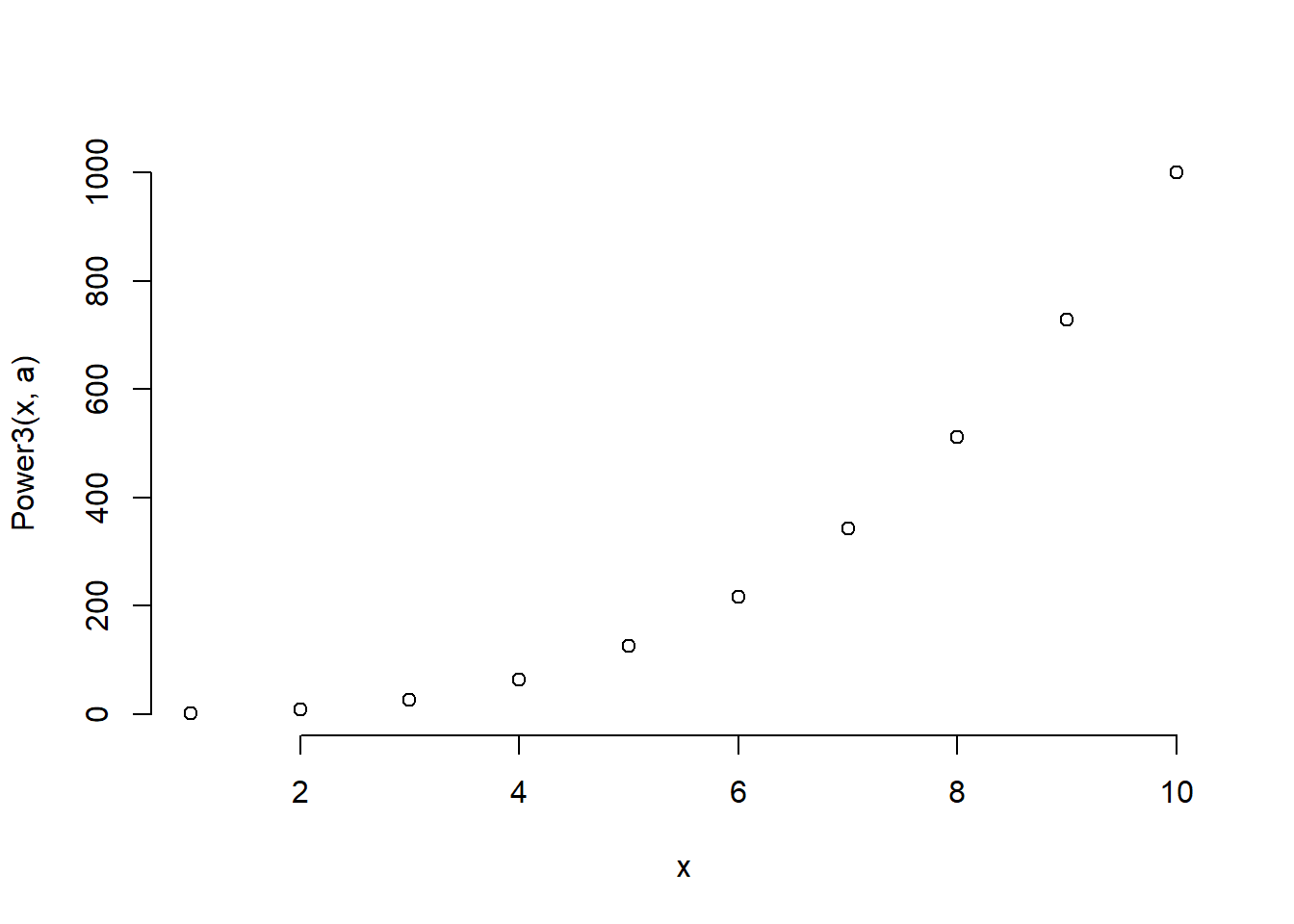You will need to load the core library for the course textbook:

# clear workspace
rm(list=ls())

library(ISLR)

#### Q1

In this problem, you will develop a model to predict whether a given car gets high or low gas mileage based on the Auto dataset from the ISLR package.

1. Create a binary variable, mpg01, that contains a 1 if mpg contains a value above its median, and a 0 if mpg contains a value below its median. You can compute the median using the median() function. Note you may find it helpful to use the data.frame() function to create a single data set containing both mpg01 and the other Auto variables. Type ?Auto to get the codebook of the dataset.
library(ISLR)
summary(Auto)
##       mpg          cylinders      displacement     horsepower
##  Min.   : 9.00   Min.   :3.000   Min.   : 68.0   Min.   : 46.0
##  1st Qu.:17.00   1st Qu.:4.000   1st Qu.:105.0   1st Qu.: 75.0
##  Median :22.75   Median :4.000   Median :151.0   Median : 93.5
##  Mean   :23.45   Mean   :5.472   Mean   :194.4   Mean   :104.5
##  3rd Qu.:29.00   3rd Qu.:8.000   3rd Qu.:275.8   3rd Qu.:126.0
##  Max.   :46.60   Max.   :8.000   Max.   :455.0   Max.   :230.0
##
##      weight      acceleration        year           origin
##  Min.   :1613   Min.   : 8.00   Min.   :70.00   Min.   :1.000
##  1st Qu.:2225   1st Qu.:13.78   1st Qu.:73.00   1st Qu.:1.000
##  Median :2804   Median :15.50   Median :76.00   Median :1.000
##  Mean   :2978   Mean   :15.54   Mean   :75.98   Mean   :1.577
##  3rd Qu.:3615   3rd Qu.:17.02   3rd Qu.:79.00   3rd Qu.:2.000
##  Max.   :5140   Max.   :24.80   Max.   :82.00   Max.   :3.000
##
##                  name
##  ford pinto        :  5
##  toyota corolla    :  5
##  amc gremlin       :  4
##  amc hornet        :  4
##  chevrolet chevette:  4
##  (Other)           :365
attach(Auto)
## The following objects are masked from Auto (pos = 22):
##
##     acceleration, cylinders, displacement, horsepower, mpg, name,
##     origin, weight, year
mpg01 <- ifelse( mpg > median(mpg), yes = 1, no = 0)
Auto <- data.frame(Auto, mpg01)
1. Explore the data graphically in order to investigate the association between mpg01 and the other features. Which of the other features seem most likely to be useful in predicting mpg01? Scatterplots and boxplots may be useful tools to answer this question. Describe your findings.
cor(Auto[,-9])
##                     mpg  cylinders displacement horsepower     weight
## mpg           1.0000000 -0.7776175   -0.8051269 -0.7784268 -0.8322442
## cylinders    -0.7776175  1.0000000    0.9508233  0.8429834  0.8975273
## displacement -0.8051269  0.9508233    1.0000000  0.8972570  0.9329944
## horsepower   -0.7784268  0.8429834    0.8972570  1.0000000  0.8645377
## weight       -0.8322442  0.8975273    0.9329944  0.8645377  1.0000000
## acceleration  0.4233285 -0.5046834   -0.5438005 -0.6891955 -0.4168392
## year          0.5805410 -0.3456474   -0.3698552 -0.4163615 -0.3091199
## origin        0.5652088 -0.5689316   -0.6145351 -0.4551715 -0.5850054
## mpg01         0.8369392 -0.7591939   -0.7534766 -0.6670526 -0.7577566
##              acceleration       year     origin      mpg01
## mpg             0.4233285  0.5805410  0.5652088  0.8369392
## cylinders      -0.5046834 -0.3456474 -0.5689316 -0.7591939
## displacement   -0.5438005 -0.3698552 -0.6145351 -0.7534766
## horsepower     -0.6891955 -0.4163615 -0.4551715 -0.6670526
## weight         -0.4168392 -0.3091199 -0.5850054 -0.7577566
## acceleration    1.0000000  0.2903161  0.2127458  0.3468215
## year            0.2903161  1.0000000  0.1815277  0.4299042
## origin          0.2127458  0.1815277  1.0000000  0.5136984
## mpg01           0.3468215  0.4299042  0.5136984  1.0000000
pairs(Auto) # doesn't work well since mpg01 is 0 or 1cylinders, weight, displacement, horsepower (and mpg itself)

1. Split the data into a training set and a test set.

First, we normalize our varibles

Auto <- data.frame(mpg01, apply(cbind(cylinders, weight, displacement, horsepower, acceleration),
2, scale), year)

We use the %% operator to split the data set into observations with even and uneven years. Check here for a description of different R operators.

train <-  (year %% 2 == 0) # if the year is even (%%)
test <-  !train
Auto.train <-  Auto[train,]
Auto.test <-  Auto[test,]
mpg01.test <-  mpg01[test]
1. Perform LDA on the training data in order to predict mpg01 using the variables that seemed most associated with mpg01 in (b). What is the test error of the model obtained?
# LDA
library(MASS)
lda.fit <-  lda(mpg01 ~ cylinders + weight + displacement + horsepower,
data = Auto, subset = train)
lda.pred <-  predict(lda.fit, Auto.test)
mean(lda.pred\$class != mpg01.test)
##  0.1263736

12.6% test error rate.

1. Perform logistic regression on the training data in order to predict mpg01 using the variables that seemed most associated with mpg01 in (b). What is the test error of the model obtained?
# Logistic regression
glm.fit <-  glm(mpg01 ~ cylinders + weight + displacement + horsepower,
data = Auto,
family = binomial,
subset = train)
glm.probs <-  predict(glm.fit, Auto.test, type = "response")
glm.pred <-  rep(0, length(glm.probs))
glm.pred[glm.probs > 0.5] <- 1
mean(glm.pred != mpg01.test)
##  0.1208791

12.1% test error rate.

1. Perform KNN on the training data, with several values of K, in order to predict mpg01. Use only the variables that seemed most associated with mpg01 in (b). What test errors do you obtain? Which value of K seems to perform the best on this data set?
library(class)
train.X <-  cbind(cylinders, weight, displacement, horsepower)[train,]
test.X <-  cbind(cylinders, weight, displacement, horsepower)[test,]
train.mpg01 <-  mpg01[train]
set.seed(1)

# KNN (k=1)
knn.pred <-  knn(train.X, test.X, train.mpg01, k = 1)
mean(knn.pred != mpg01.test)
##  0.1538462
# KNN (k=10)
knn.pred <-  knn(train.X, test.X, train.mpg01, k = 10)
mean(knn.pred != mpg01.test)
##  0.1648352
# KNN (k=100)
knn.pred <-  knn(train.X, test.X, train.mpg01, k = 100)
mean(knn.pred != mpg01.test)
##  0.1428571

k=1, 15.4% test error rate. k=10, 16.5% test error rate. k=100, 14.3% test error rate. K of 100 seems to perform the best. 100 nearest neighbors.

1. We can find the optimal value for K by trying all K from 1 to 200. We will run a loop and save the test error for every value of K.
K.collector <- rep(NA, 200)
for (k.try in 1:200){
knn.pred <-  knn(train.X, test.X, train.mpg01, k = k.try)
K.collector[k.try] <- mean(knn.pred != mpg01.test)
}
x.k <- c(1:200)

plot(x.k,K.collector, type="o", pch=19, cex=0.5, main="K ")It is hard to see the optimal value of K for us. We can inspect the error ourselves:

which.min(K.collector)
##  4

Here, it seems to be the case that K=4 is the best. We will see tomorrow how we can ensure that this does not just depend on the specific trianing and test data we created here.

#### Q2

This problem involves writing functions.

1. Write a function, Power(), that prints out the result of raising 2 to the 3rd power. In other words, your function should compute $$2^3$$ and print out the results.

Hint: Recall that $$x^a$$ raises x to the power a. Use the print() function to output the result.

Power <-  function() {
2^3
}
print(Power())
##  8
1. Create a new function, Power2(), that allows you to pass any two numbers, x and a, and prints out the value of x^a. You can do this by beginning your function with the line:
Power2 <- function(x,a) {

You should be able to call your function by entering, for instance,

Power2(3,8)

on the command line. This should output the value of $$3^8$$, namely, 6,561.

Power2 <-  function(x, a) {
x^a
}
Power2(3,8)
##  6561
1. Using the Power2() function that you just wrote, compute $$10^3$$, $$8^{17}$$, and $$131^3$$.
Power2(10, 3)
##  1000
Power2(8, 17)
##  2.2518e+15
Power2(131, 3)
##  2248091
1. Now create a new function, Power3(), that actually returns the result x^a as an R object, rather than simply printing it to the screen. That is, if you store the value x^a in an object called result within your function, then you can simply return() this result, using the following line:
return(result)

The line above should be the last line in your function, before the } symbol.

Power3 <-  function(x, a) {
result <-  x^a
return(result)
}
1. Now using the Power3() function, create a plot of $$f(x)=x^2$$. The $$x$$-axis should display a range of integers from 1 to 10, and the $$y$$-axis should display $$x^2$$. Label the axes appropriately, and use an appropriate title for the figure. Consider displaying either the $$x$$-axis, the $$y$$-axis, or both on the log-scale. You can do this by using log="x", log="y", or log="xy" as arguments to the plot() function.
x <-  1:10
plot(x, Power3(x, 2),
log="xy", ylab="Log of y = x^2", xlab="Log of x",
main="Log of x^2 versus Log of x", bty = "n")1. Create a function, PlotPower(), that allows you to create a plot of x against x^a for a fixed a and for a range of values of x. For instance, if you call
PlotPower(1:10, 3)

then a plot should be created with an $$x$$-axis taking on values $$1,2,...,10$$, and a $$y$$-axis taking on values $$1^3,2^3,...,10^3$$.

PlotPower <-  function(x, a) {
plot(x, Power3(x, a), bty = "n")
}
PlotPower(1:10, 3)